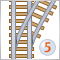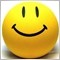3rd Period of RSI19

Hello everyone;

Would anyone please try to run this RSI file posted down here using InputPeriodRSI = 3 on different currency pairs and different time-frames per each currency pair.

This file is posted by MetaQuotes, so I initially assume that this is the file they use to calculate the RSI.

Guys, I don't know why for this Period ONLY number (3) only gives me problems drawing it !!

The code does work thou on M1 & M5, and some times on MN & W. but for example the M30, M20 or H1, H4, H6 Don't wanna plot the RSI(3) for that time-frame for this currency pair.

Unfortunately I need this RSI(3) specifically with that code because I'm working on certain indicator that needs this RSI(3) calculations from this code!

The Code works perfect on all other RSI periods for all the different currencies with all time-frames. But I don't know why specifically it gives me darn hard time on the 3rd period that I badly need for this custom indicator.

Thanx everyone to consider the time to read & look @ my problem :)

-- PipCaesar

//+------------------------------------------------------------------+
//|                                                          RSI.mq5 |
//|                        Copyright 2009, MetaQuotes Software Corp. |
//|                                              http://www.mql5.com |
//+------------------------------------------------------------------+
#property copyright   "2009, MetaQuotes Software Corp."
#property description "Relative Strength Index"
//--- indicator settings
#property indicator_separate_window
#property indicator_minimum 0
#property indicator_maximum 100
#property indicator_level1 30
#property indicator_level2 70
#property indicator_buffers 3
#property indicator_plots   1
#property indicator_type1   DRAW_LINE
#property indicator_color1  DodgerBlue
//--- input parameters
input int InpPeriodRSI=3; // Period
//--- indicator buffers
double    ExtRSIBuffer[];
double    ExtPosBuffer[];
double    ExtNegBuffer[];
//--- global variable
int       ExtPeriodRSI;
//+------------------------------------------------------------------+
//| Custom indicator initialization function                         |
//+------------------------------------------------------------------+
void OnInit()
{
//--- check for input
if(InpPeriodRSI<1)
{
ExtPeriodRSI=12;
Print("Incorrect value for input variable InpPeriodRSI =",InpPeriodRSI,
"Indicator will use value =",ExtPeriodRSI,"for calculations.");
}
else ExtPeriodRSI=InpPeriodRSI;
//--- indicator buffers mapping
SetIndexBuffer(0,ExtRSIBuffer,INDICATOR_DATA);
SetIndexBuffer(1,ExtPosBuffer,INDICATOR_CALCULATIONS);
SetIndexBuffer(2,ExtNegBuffer,INDICATOR_CALCULATIONS);
//--- set accuracy
IndicatorSetInteger(INDICATOR_DIGITS,2);
//--- sets first bar from what index will be drawn
PlotIndexSetInteger(0,PLOT_DRAW_BEGIN,ExtPeriodRSI);
//--- name for DataWindow and indicator subwindow label
IndicatorSetString(INDICATOR_SHORTNAME,"RSI("+string(ExtPeriodRSI)+")");
//--- initialization done
}
//+------------------------------------------------------------------+
//| Relative Strength Index                                          |
//+------------------------------------------------------------------+
int OnCalculate(const int rates_total,
const int prev_calculated,
const int begin,
const double &price[])
{
int    i;
double diff;
//--- check for rates count
if(rates_total<=ExtPeriodRSI)
return(0);
//--- preliminary calculations
int pos=prev_calculated-1;
if(pos<=ExtPeriodRSI)
{
//--- first RSIPeriod values of the indicator are not calculated
ExtRSIBuffer=0.0;
ExtPosBuffer=0.0;
ExtNegBuffer=0.0;
double SumP=0.0;
double SumN=0.0;
for(i=1;i<=ExtPeriodRSI;i++)
{
ExtRSIBuffer[i]=0.0;
ExtPosBuffer[i]=0.0;
ExtNegBuffer[i]=0.0;
diff=price[i]-price[i-1];
SumP+=(diff>0?diff:0);
SumN+=(diff<0?-diff:0);
}
//--- calculate first visible value
ExtPosBuffer[ExtPeriodRSI]=SumP/ExtPeriodRSI;
ExtNegBuffer[ExtPeriodRSI]=SumN/ExtPeriodRSI;
ExtRSIBuffer[ExtPeriodRSI]=100.0-(100.0/(1.0+ExtPosBuffer[ExtPeriodRSI]/ExtNegBuffer[ExtPeriodRSI]));
//--- prepare the position value for main calculation
pos=ExtPeriodRSI+1;
}
//--- the main loop of calculations
for(i=pos;i<rates_total && !IsStopped();i++)
{
diff=price[i]-price[i-1];
ExtPosBuffer[i]=(ExtPosBuffer[i-1]*(ExtPeriodRSI-1)+(diff>0.0?diff:0.0))/ExtPeriodRSI;
ExtNegBuffer[i]=(ExtNegBuffer[i-1]*(ExtPeriodRSI-1)+(diff<0.0?-diff:0.0))/ExtPeriodRSI;
ExtRSIBuffer[i]=100.0-100.0/(1+ExtPosBuffer[i]/ExtNegBuffer[i]);
}
//--- OnCalculate done. Return new prev_calculated.
return(rates_total);
}
//+------------------------------------------------------------------+Step on New Rails: Custom Indicators in MQL5
• 2009.11.23
• Андрей
• www.mql5.com
I will not list all of the new possibilities and features of the new terminal and language. They are numerous, and some novelties are worth the discussion in a separate article. Also there is no code here, written with object-oriented programming, it is a too serous topic to be simply mentioned in a context as additional advantages for developers. In this article we will consider the indicators, their structure, drawing, types and their programming details, as compared to MQL4. I hope that this article will be useful both for beginners and experienced developers, maybe some of them will find something new.2100

PipCaesar:

Hello everyone;

Would anyone please try to run this RSI file posted down here using InputPeriodRSI = 3 on different currency pairs and different time-frames per each currency pair.

This file is posted by MetaQuotes, so I initially assume that this is the file they use to calculate the RSI.

Guys, I don't know why for this Period ONLY number (3) only gives me problems drawing it !!

The code does work thou on M1 & M5, and some times on MN & W. but for example the M30, M20 or H1, H4, H6 Don't wanna plot the RSI(3) for that time-frame for this currency pair.

Unfortunately I need this RSI(3) specifically with that code because I'm working on certain indicator that needs this RSI(3) calculations from this code!

The Code works perfect on all other RSI periods for all the different currencies with all time-frames. But I don't know why specifically it gives me darn hard time on the 3rd period that I badly need for this custom indicator.

Thanx everyone to consider the time to read & look @ my problem :)

-- PipCaesar

You must be talking about RSI that MetaQuotes published in code base in here. Yes, I try the code you posted, and sometime it draw nothing at all at period 3 on all different time frame. I simply have switch to another time frame and go back again and the line then displayed properly.

There's also RSI in MT5 under Custom Indicator/Examples/RSI which I also try and gives no displaying error. You may want to try that one instead the one you posted.

The differences between RSI in MT5 and in code base is, that the RSI in code base does not have this below, which I don't know if that is the one that give the display error

//--- the main loop of calculations
for(i=pos;i<rates_total && !IsStopped();i++)
{
diff=price[i]-price[i-1];
ExtPosBuffer[i]=(ExtPosBuffer[i-1]*(ExtPeriodRSI-1)+(diff>0.0?diff:0.0))/ExtPeriodRSI;
ExtNegBuffer[i]=(ExtNegBuffer[i-1]*(ExtPeriodRSI-1)+(diff<0.0?-diff:0.0))/ExtPeriodRSI;
if(ExtNegBuffer[i]!=0.0)
ExtRSIBuffer[i]=100.0-100.0/(1+ExtPosBuffer[i]/ExtNegBuffer[i]);
else
{
if(ExtPosBuffer[i]!=0.0)
ExtRSIBuffer[i]=100.0;
else
ExtRSIBuffer[i]=50.0;
}
}19

phi.nuts:

You must be talking about RSI that MetaQuotes published in code base in here. Yes, I try the code you posted, and sometime it draw nothing at all at period 3 on all different time frame. I simply have switch to another time frame and go back again and the line then displayed properly.

There's also RSI in MT5 under Custom Indicator/Examples/RSI which I also try and gives no displaying error. You may want to try that one instead the one you posted.

The differences between RSI in MT5 and in code base is, that the RSI in code base does not have this below, which I don't know if that is the one that give the display error

Phi.nuts, Hello to u;

Thanx greatly for attracting my attention to that NEW (for me) code of the RSI.

I'll work on it today.

Thanx again.

-- PipCaesar17730

PipCaesar:

Hello everyone;

Would anyone please try to run this RSI file posted down here using InputPeriodRSI = 3 on different currency pairs and different time-frames per each currency pair.

This file is posted by MetaQuotes, so I initially assume that this is the file they use to calculate the RSI.

Guys, I don't know why for this Period ONLY number (3) only gives me problems drawing it !!

The code does work thou on M1 & M5, and some times on MN & W. but for example the M30, M20 or H1, H4, H6 Don't wanna plot the RSI(3) for that time-frame for this currency pair.

Unfortunately I need this RSI(3) specifically with that code because I'm working on certain indicator that needs this RSI(3) calculations from this code!

The Code works perfect on all other RSI periods for all the different currencies with all time-frames. But I don't know why specifically it gives me darn hard time on the 3rd period that I badly need for this custom indicator.

Why do you need 2 threads to discuss the same subject ?  https://www.mql5.com/en/forum/10931